# Evolutionary Programming Chapter 5 Evolutionary Programming A E

• Slides: 18
Download presentation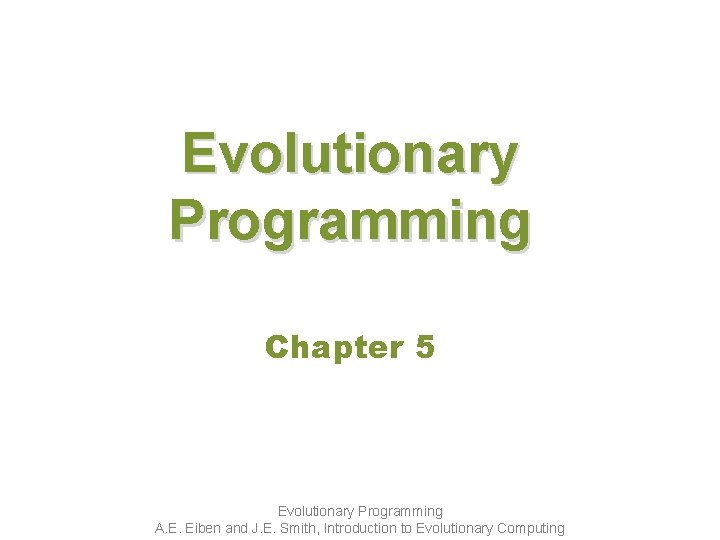Evolutionary Programming Chapter 5 Evolutionary Programming A. E. Eiben and J. E. Smith, Introduction to Evolutionary Computing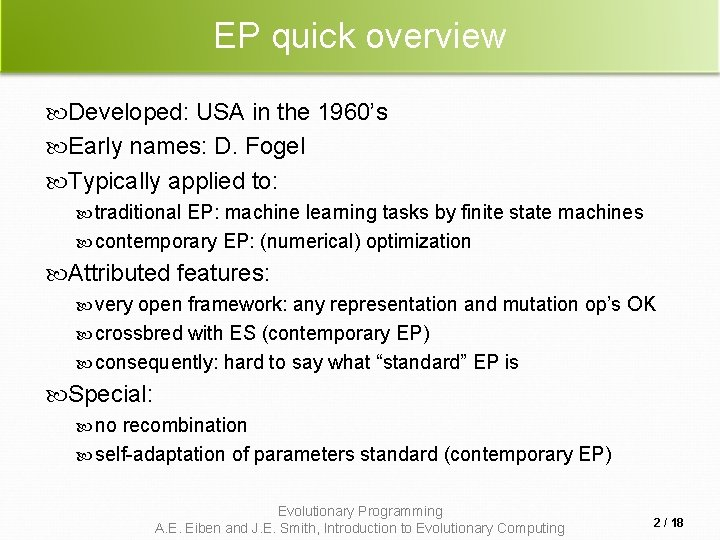EP quick overview Developed: USA in the 1960’s Early names: D. Fogel Typically applied to: traditional EP: machine learning tasks by finite state machines contemporary EP: (numerical) optimization Attributed features: very open framework: any representation and mutation op’s OK crossbred with ES (contemporary EP) consequently: hard to say what “standard” EP is Special: no recombination self-adaptation of parameters standard (contemporary EP) Evolutionary Programming A. E. Eiben and J. E. Smith, Introduction to Evolutionary Computing 2 / 18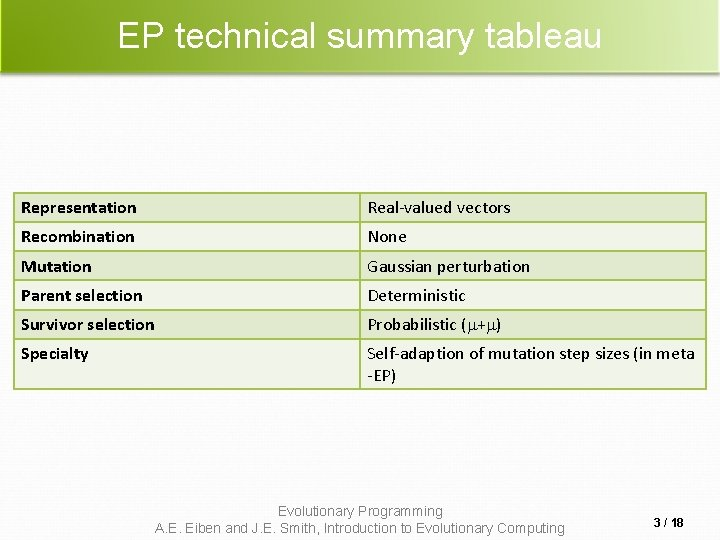EP technical summary tableau Representation Real-valued vectors Recombination None Mutation Gaussian perturbation Parent selection Deterministic Survivor selection Probabilistic ( + ) Specialty Self-adaption of mutation step sizes (in meta -EP) Evolutionary Programming A. E. Eiben and J. E. Smith, Introduction to Evolutionary Computing 3 / 18Historical EP perspective EP aimed at achieving intelligence Intelligence was viewed as adaptive behaviour Prediction of the environment was considered a prerequisite to adaptive behaviour Thus: capability to predict is key to intelligence Evolutionary Programming A. E. Eiben and J. E. Smith, Introduction to Evolutionary Computing 4 / 18Prediction by finite state machines Finite state machine (FSM): States S Inputs I Outputs O Transition function : S x I S x O Transforms input stream into output stream Can be used for predictions, e. g. to predict next input symbol in a sequence Evolutionary Programming A. E. Eiben and J. E. Smith, Introduction to Evolutionary Computing 5 / 18FSM example Consider the FSM with: S = {A, B, C} I = {0, 1} O = {a, b, c} given by a diagram Evolutionary Programming A. E. Eiben and J. E. Smith, Introduction to Evolutionary Computing 6 / 18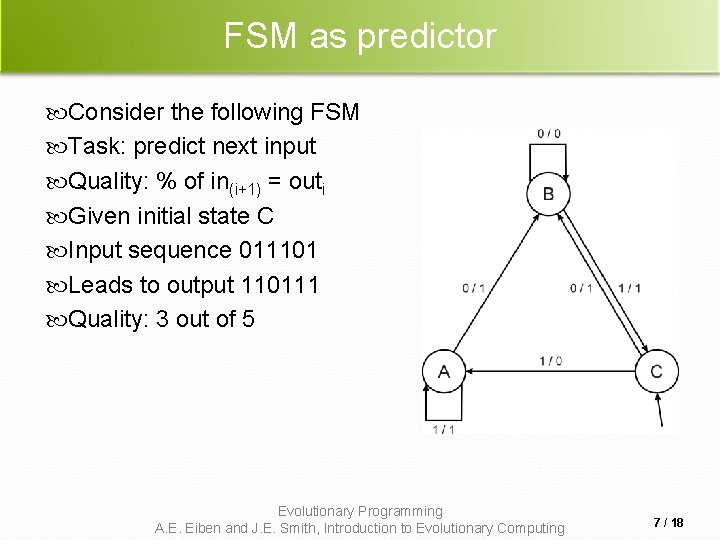FSM as predictor Consider the following FSM Task: predict next input Quality: % of in(i+1) = outi Given initial state C Input sequence 011101 Leads to output 110111 Quality: 3 out of 5 Evolutionary Programming A. E. Eiben and J. E. Smith, Introduction to Evolutionary Computing 7 / 18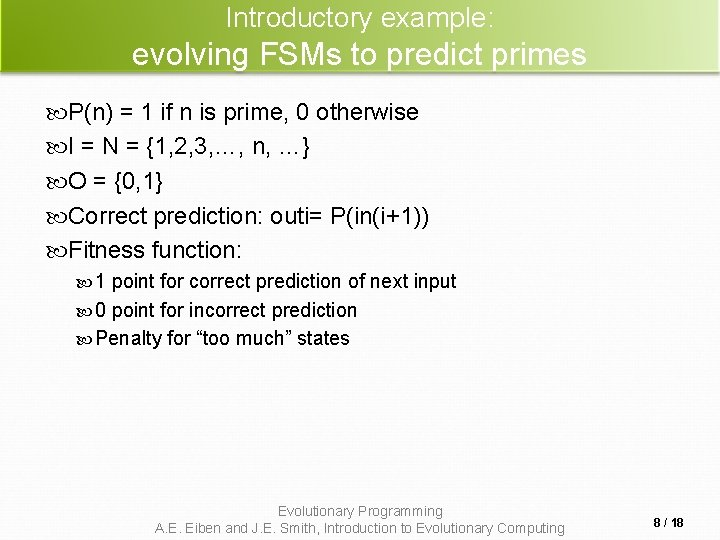Introductory example: evolving FSMs to predict primes P(n) = 1 if n is prime, 0 otherwise I = N = {1, 2, 3, …, n, …} O = {0, 1} Correct prediction: outi= P(in(i+1)) Fitness function: 1 point for correct prediction of next input 0 point for incorrect prediction Penalty for “too much” states Evolutionary Programming A. E. Eiben and J. E. Smith, Introduction to Evolutionary Computing 8 / 18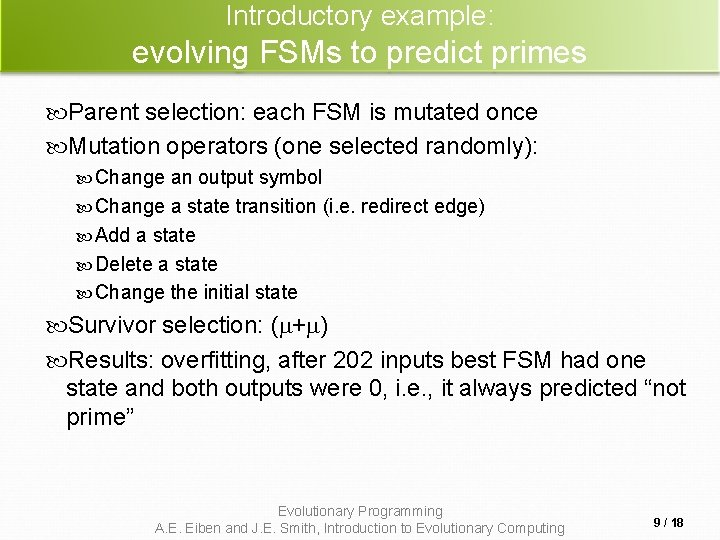Introductory example: evolving FSMs to predict primes Parent selection: each FSM is mutated once Mutation operators (one selected randomly): Change an output symbol Change a state transition (i. e. redirect edge) Add a state Delete a state Change the initial state Survivor selection: ( + ) Results: overfitting, after 202 inputs best FSM had one state and both outputs were 0, i. e. , it always predicted “not prime” Evolutionary Programming A. E. Eiben and J. E. Smith, Introduction to Evolutionary Computing 9 / 18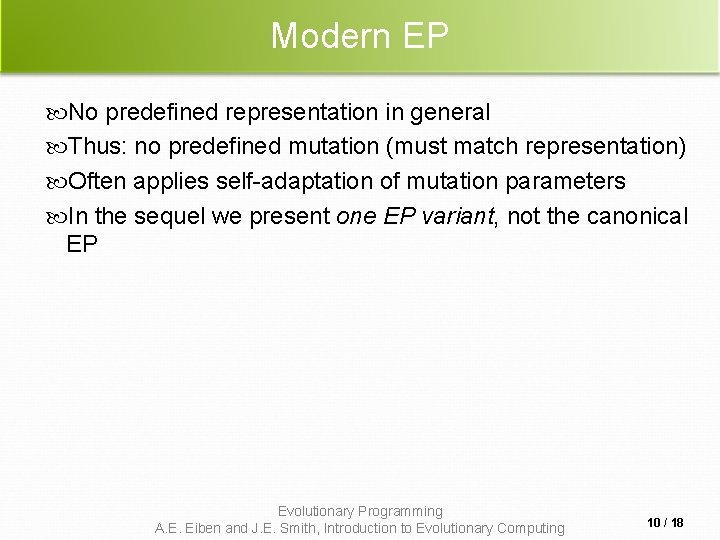Modern EP No predefined representation in general Thus: no predefined mutation (must match representation) Often applies self-adaptation of mutation parameters In the sequel we present one EP variant, not the canonical EP Evolutionary Programming A. E. Eiben and J. E. Smith, Introduction to Evolutionary Computing 10 / 18Representation For continuous parameter optimisation Chromosomes consist of two parts: Object variables: x 1, …, xn Mutation step sizes: 1, …, n Full size: x 1, …, xn, 1, …, n Evolutionary Programming A. E. Eiben and J. E. Smith, Introduction to Evolutionary Computing 11 / 18Mutation Chromosomes: x 1, …, xn, 1, …, n i’ = i • (1 + • N(0, 1)) xi’ = xi + i’ • Ni(0, 1) 0. 2 boundary rule: ’ < 0 ’ = 0 Other variants proposed & tried: Lognormal scheme as in ES Using variance instead of standard deviation Mutate -last Other distributions, e. g, Cauchy instead of Gaussian Evolutionary Programming A. E. Eiben and J. E. Smith, Introduction to Evolutionary Computing 12 / 18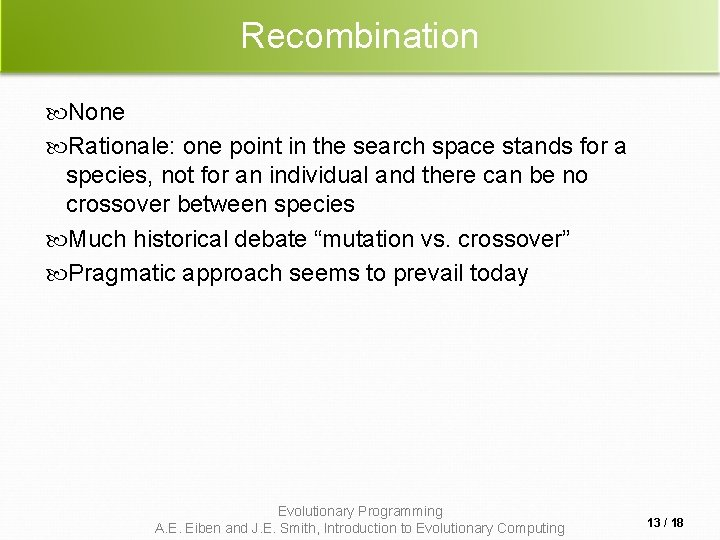Recombination None Rationale: one point in the search space stands for a species, not for an individual and there can be no crossover between species Much historical debate “mutation vs. crossover” Pragmatic approach seems to prevail today Evolutionary Programming A. E. Eiben and J. E. Smith, Introduction to Evolutionary Computing 13 / 18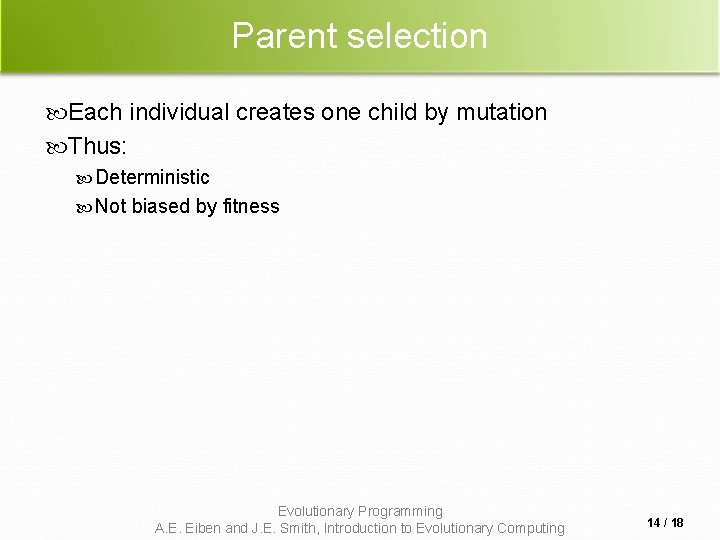Parent selection Each individual creates one child by mutation Thus: Deterministic Not biased by fitness Evolutionary Programming A. E. Eiben and J. E. Smith, Introduction to Evolutionary Computing 14 / 18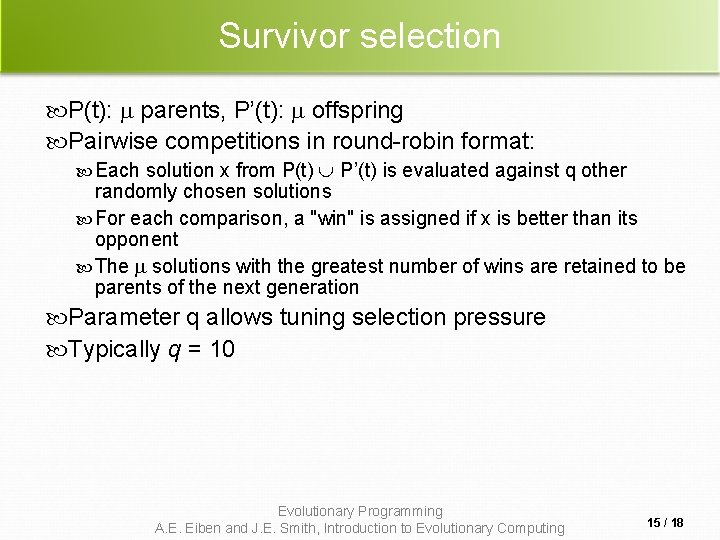Survivor selection P(t): parents, P’(t): offspring Pairwise competitions in round-robin format: Each solution x from P(t) P’(t) is evaluated against q other randomly chosen solutions For each comparison, a "win" is assigned if x is better than its opponent The solutions with the greatest number of wins are retained to be parents of the next generation Parameter q allows tuning selection pressure Typically q = 10 Evolutionary Programming A. E. Eiben and J. E. Smith, Introduction to Evolutionary Computing 15 / 18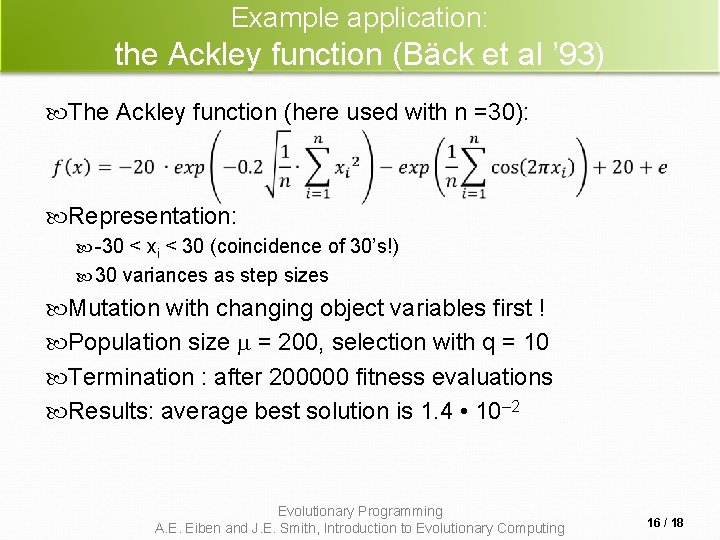Example application: the Ackley function (Bäck et al ’ 93) The Ackley function (here used with n =30): Representation: -30 < xi < 30 (coincidence of 30’s!) 30 variances as step sizes Mutation with changing object variables first ! Population size = 200, selection with q = 10 Termination : after 200000 fitness evaluations Results: average best solution is 1. 4 • 10– 2 Evolutionary Programming A. E. Eiben and J. E. Smith, Introduction to Evolutionary Computing 16 / 18Example application: evolving checkers players (Fogel’ 02) Neural nets for evaluating future values of moves are evolved NNs have fixed structure with 5046 weights, these are evolved + one weight for “kings” Representation: vector of 5046 real numbers for object variables (weights) vector of 5046 real numbers for ‘s Mutation: Gaussian, lognormal scheme with -first Plus special mechanism for the kings’ weight Population size 15 Evolutionary Programming A. E. Eiben and J. E. Smith, Introduction to Evolutionary Computing 17 / 18Example application: evolving checkers players (Fogel’ 02) Tournament size q = 5 Programs (with NN inside) play against other programs, no human trainer or hard-wired intelligence After 840 generation (6 months!) best strategy was tested against humans via Internet Program earned “expert class” ranking outperforming 99. 61% of all rated players Evolutionary Programming A. E. Eiben and J. E. Smith, Introduction to Evolutionary Computing 18 / 18